# Catch up on the latest AI articles# Ultra-lightweight CNN Speech Recognition Model! Google-developed "ContextNet" Explained!

3 main points
✔️ Google proposes a lightweight CNN speech recognition model
✔️ Consider global context with squeeze-and-excitation module
✔️ Reduce computing costs with Progressive Downsampling

written by Wei HanZhengdong ZhangYu ZhangJiahui YuChung-Cheng ChiuJames QinAnmol GulatiRuoming PangYonghui Wu
(Submitted on 7 May 2020 (v1), last revised 16 May 2020 (this version, v3))

Subjects: Audio and Speech Processing (eess.AS); Computation and Language (cs.CL); Machine Learning (cs.LG); Sound (cs.SD)code：The images used in this article are from the paper or created based on it.

## first of all

There is a growing interest in building E2E (End-to-End) speech recognition models based on CNNs instead of RNNs or Transformers. Although RNN and Transformer-based methods tend to achieve high accuracy in speech recognition, they are prone to a huge number of parameters, which results in very high computing costs.

On the other hand, CNN-based models are more parameter efficient than RNNs and transformers, which may make it easier for small companies to produce high-quality speech recognition models in practice.

However, CNN models are good at convolutional consideration of close features, but not good at considering distant global contexts, and even QuartzNet, the CNN model of SOTA, is not as accurate as RNN/Transformer models.

In such a situation, ContextNet, which we introduce here, achieves global context consideration by squeeze-and-excitation and parameter reduction by progressive downsampling simultaneously. Despite being a CNN-based model, the accuracy of ContextNet exceeds that of Transformer and LSTM-based models. The figure below shows the trade-off between model size and accuracy ( WER ) and shows that ContextNet has the best trade-off performance over AuartzNet and RNN/Transformer-based models.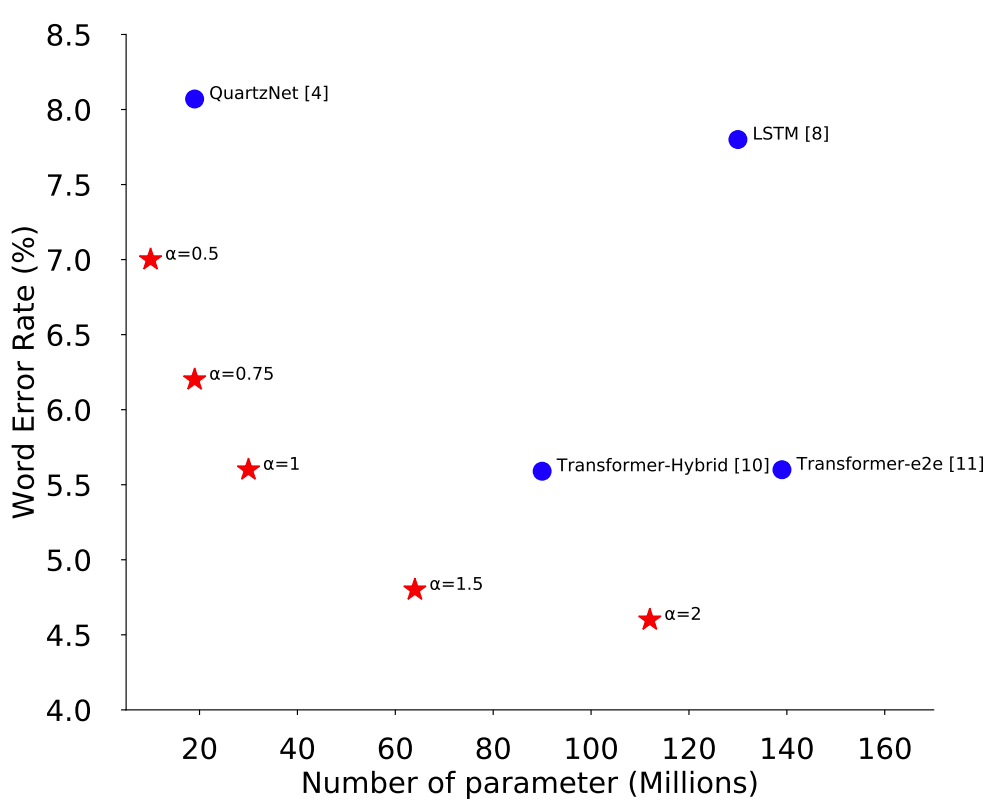Now, let's take a look at the details of the ContextNet model.

## model

### End-to-end Network: CNN-RNN-Transducer

The ContextNet network is based on the RNN-Transducer framework ) and consists of an Audio Encoder for input audio, a Label Encoder for input labels, and a The ContextNet network is based on the RNN-Transducer framework (). In this method, the Audio Encoder is changed to a CNN-based one, which is a new point of our proposal.

### Encoder Design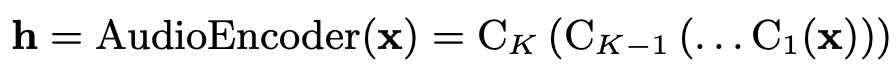Each Ck(-) is a convolution block, consisting of several convolution layers with batch normalization and activation functions after it. It also has a squeeze-and-excitation and skip connection.

Before going into a detailed description of C(-), let's start with the important modules of C(- ).

### Squeeze-and-excitation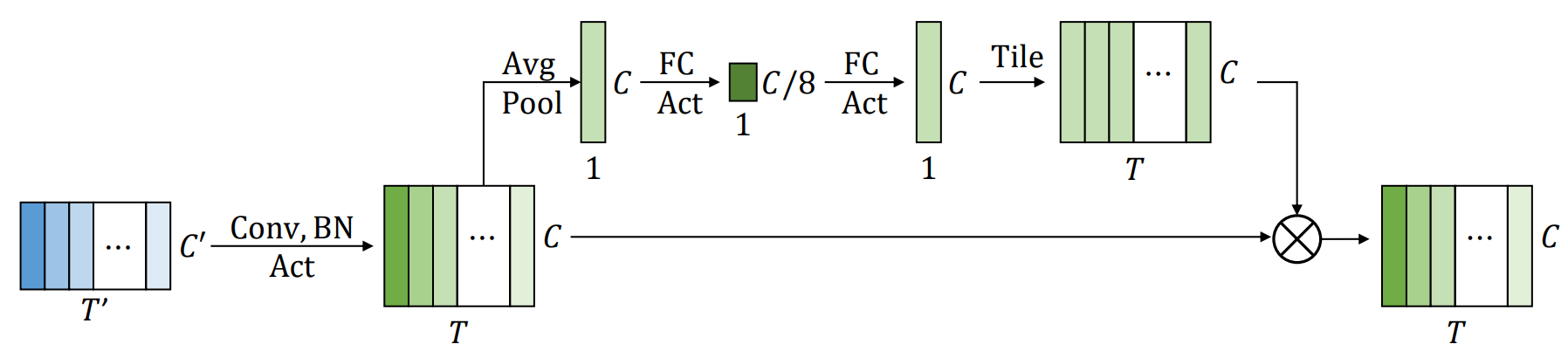As shown in the figure above, the squeeze-and-excitation function SE(-) performs global average pooling on the input x, converts it to a global channel-wise weight θ(x ), and then takes the element-wise multiplication of each frame based on this weight. Based on this weight, it takes the element-wise multiplication of each frame. Applying this idea to the 1D case, we obtain the following equation.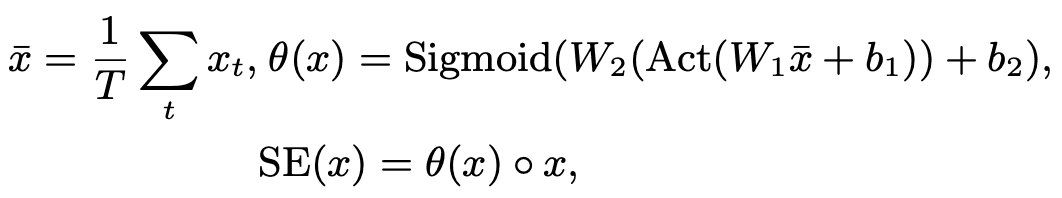The following code shows how to do this in TensorFlow. It is very simple, so please try to install the squeeze-and-excitation module in your model.

x_orig = x

x = tf.reduce_sum(x, axis=1) / tf.expand_dims(tf.cast(x_len, tf.float32), 1) # Average Pooling

for i in rage(len(num_units)):

x = tf.nn.swish(fc_layers[i][x]))

x = tf.expand_dims(tf.nn.sigmoid(x), 1)

return x * x_orig

### Depthwise separable convolution

To achieve higher parameter efficiency without sacrificing performance, we use depthwise separable convolution instead of just convolution. conv(-) denotes depthwise separable convolution.

By the way, depthwise separable convolution is a technique used in MobileNet, which is known for lightweight models. The same processing can be achieved with fewer parameters.

The code for depthwise convolution by Tensorflow is as follows.

conv = tf.keras.layers.Separable1D(filters, kernel_size, strides, padding)

### Swish Activation Function

Act(-) denotes the activation function. We tried two activation functions, ReLU and Swish, and found that Swish performs better than ReLU; the equation and graph of Swish are shown below. The derivative of the Swish function varies more smoothly than the ReLU function, which is discrete between 0 and 1, so the training results are also smoother.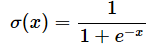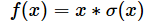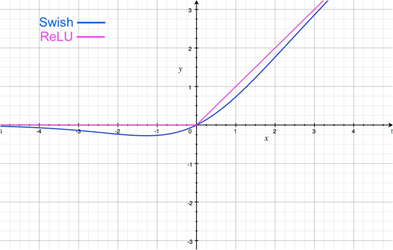In Tensorflow, it can be implemented as follows.

X = tf.nn.swish(x)

### Convolution block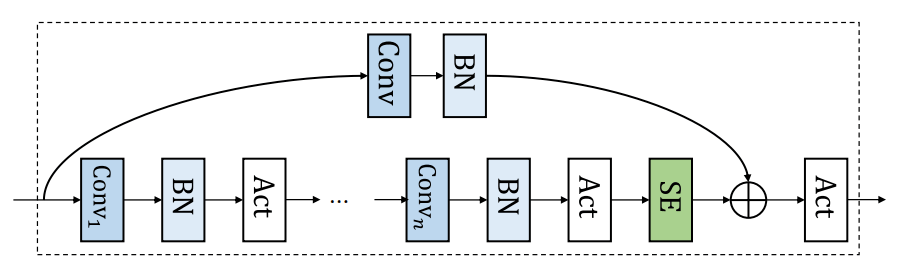The individual modules introduced above can be combined as shown in the figure above. In addition, the following is the expression of C(-) in the first equation.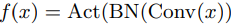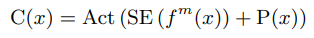where f^m is the stacked m-layerf(-), and P(-) represents pointwise projection on the residual. The code for this part of the process is as follows.

for conv_layer in conv_layers:

x = conv_layer(x)

x = se_layer(x)

x = x + residual(x_orig)

x = tf.nn.swish(x)

### Progressive downsampling

To further reduce the computing cost, we employ progressive downsampling. Specifically, we experiment by gradually increasing the stride of the convolutional layer and observe the trade-off between the number of parameters and the performance. As a result, the best trade-off result is obtained when downsampling with 8x for ContextNet.

### Configuration details of ContextNet

ContextNet consists of 23 convolutional blocks ( C0, ..., C22 ), and all but C0 and C22 have 5 convolutional layers. The following figure is a summary of the architectural details.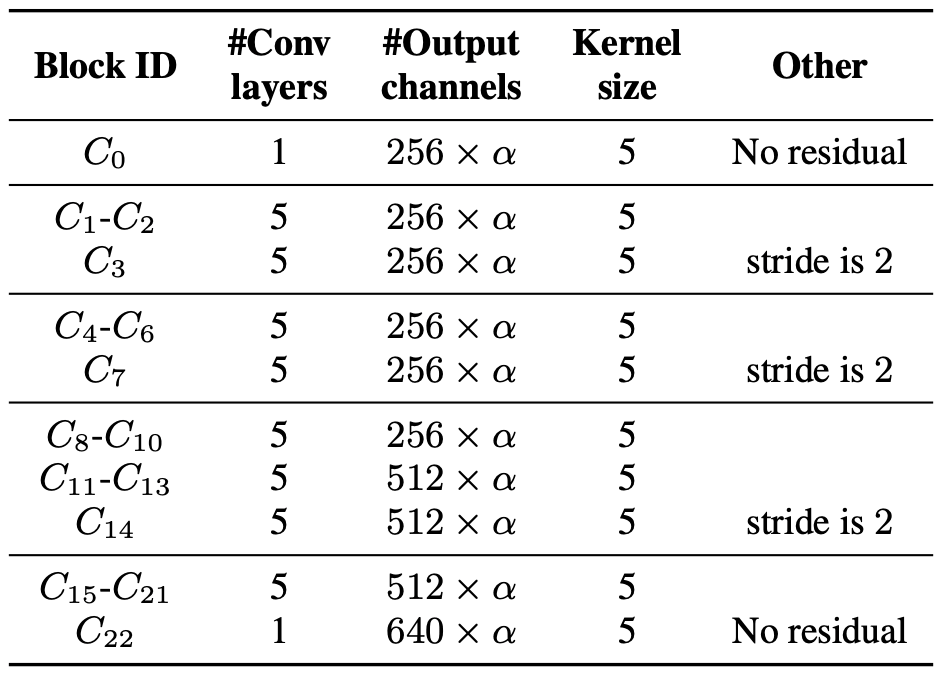Here, the global parameter α controls the scaling of the model and increasing it to α > 1 increases the number of convolution channels.

## experimental results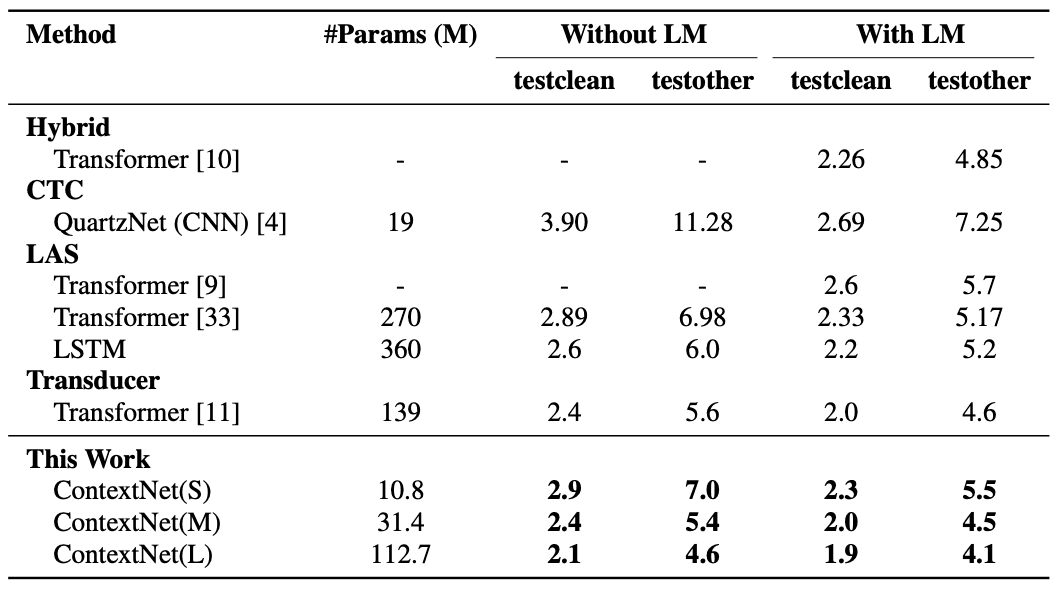The above figure shows the WER values when tested on the Librispeech dataset. Also from the above figure. We can see that ContextNet outperforms the other models in both cases, with and without the language model ( LM).

In the figure below, you can also see that the squeeze-and-excitation module greatly improves the performance by considering the global context.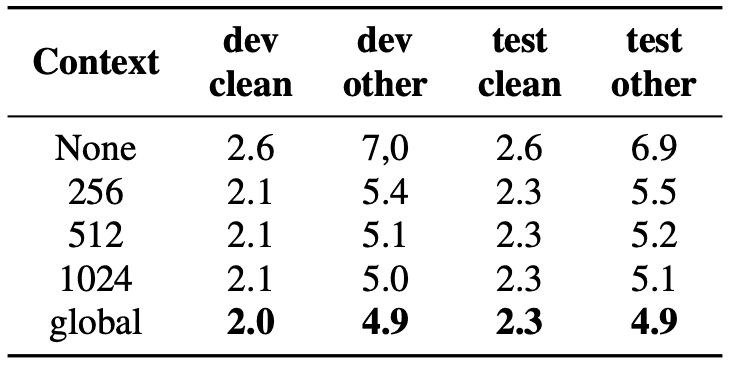## Finally.

In this article, we introduced a CNN-based end-to-end speech recognition model " ContextNet ". Although transformers are commonly used nowadays, their computing cost is very high, and it is difficult for individuals and small companies to afford them. On the other hand, CNN-based transformers can be implemented at a relatively low cost as shown in this paper, so why don't you try using techniques such as squeeze-and-excitation and progressive downsampling?

If you have any suggestions for improvement of the content of the article,